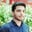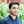Trusted answers to developer questions
Trusted Answers to Developer Questions

Related Tags

numpy
python

# What is numpy.left_shift() in Python?Salman Yousaf

Grokking Modern System Design Interview for Engineers & Managers

Ace your System Design Interview and take your career to the next level. Learn to handle the design of applications like Netflix, Quora, Facebook, Uber, and many more in a 45-min interview. Learn the RESHADED framework for architecting web-scale applications by determining requirements, constraints, and assumptions before diving into a step-by-step design process.

## Overview

The numpy.left_shift() method is used to shift bits to the left x2 times by appending 0s at the right of x1.

Note: The left shift operation is equivalent to the multiplying x1 by $2^2$.

### Syntax

numpy.left_shift(arr1,
arr2,
/,
out=None,
*,
where=True,
casting='same_kind',
order='K',
dtype=None,
ufunc 'left_shift')

### Parameters

It takes the following argument values.

• arr1: Integer number or array_like instance.
• arr2: Integer number or array_like instance. It shows the number of 0s which will be appended to x1. If x1.shape is not equal to x2.shape, then its dimensions should be able to be broadcasted.
• out: A location where results are stored. It can be ndarray, None, and a tuple of ndarray. Its default value is None.
• where: Array-like instance. It shows a condition where broadcasting is applied. Its default value is True.
• **kwargs: The other arguments are optional and show keyword argument values.

### Return value

It returns either a scalar value or an integer array.

• out: It returns x1 with x2 times a left-shifted number.

### Case 1: Where x1 & x2 are both scalar values

# Case#1 where x1 & x2 both are scalar values.# loading numpy libraryimport numpy as np# defining x1 and x2 numbersx1= 10x2= 2# invoking left_shift() method to perform left shift operationout= np.left_shift(x1, x2)# print output on console.print("After left shifting 2 bit : ", out)
Applying left_shift() on two scalar values
• Line 5–6: We define x1 and x2 as integer values.
• Line 8: We call left_shift() with x1 as 10 and x2 as 2 to left shift x1 by 2 times. It prints 40 because the left shift is also equivalent to $10*2^2$.
• Line 10: We print the left-shifted number to the console.

### Case 2: Where x1 is a scalar while x2 is an integer array

# Case#2 where x1 is scalar while x2 is integer array# loading numpy libraryimport numpy as np# defining an integer as well as integer arrayx1= 5x2= [1, 2, 3]# print numbers on consoleprint("Input number : ", x1)print("Number of bit shift : ", x2)# left x1, x2 timesout= np.left_shift(x1, x2)# print numbers on consoleprint("Output array after left shifting: ", out)
Applying left_shift() on one scalar value and an array
• Line 5–6: We define x1 as5 (scalar) while we define x2 as [1, 2, 3] (integer array).
• Line 8–9: We print x1 as well as x2 to the console.
• Line 11: The np.left_shift(x1, x2) statement shifts x1 values, x2 times at each index of arrays.
• Line 13: We print the left-shifted number to the console.

### Case 3: Where x1 & x2 are both integer arrays

# Case#3 where x1 & x2 both are integer arrays.# loading numpy libraryimport numpy as np# defining two integer arraysx1= [2, 8, 15]x2= [3, 4, 5]# shows above arrays on consoleprint("Input array : ", x1)print("Number of bit shift : ", x2)# invoking left_shift() to shift x1, x2 timesout= np.left_shift(x1, x2)# results on the consoleprint("Output array after left shifting: ", out)
Applying left_shift() on two arrays
• Line 5–6: We define x1 as well as x2 as two integer arrays.
• Line 8–9: We print the x1 and x2 arrays to the console.
• Line 11: The np.left_shift(x1, x2) statement will left shift x1 array values x2 times each time the number parallel to each index.
• Line 13: We print the left-shifted results as integer array to the console: [ 16 128 480].

RELATED TAGS

numpy
python

CONTRIBUTORSalman Yousaf

Grokking Modern System Design Interview for Engineers & Managers

Ace your System Design Interview and take your career to the next level. Learn to handle the design of applications like Netflix, Quora, Facebook, Uber, and many more in a 45-min interview. Learn the RESHADED framework for architecting web-scale applications by determining requirements, constraints, and assumptions before diving into a step-by-step design process.

Keep Exploring

Learn in-demand tech skills in half the time